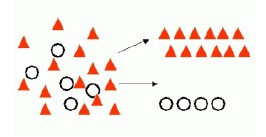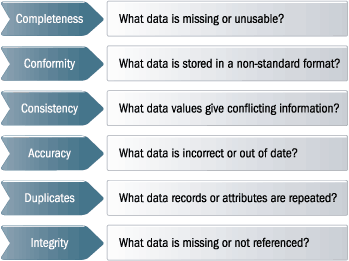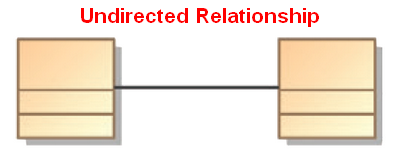# Distance

Distance is a numerical description of how far apart objects are.

Same as:

In most cases, “distance from A to B” is interchangeable with “distance between B and A”.

## Definition

### Physics

In physics or everyday usage, distance may refer to a physical length, or an estimation based on other criteria (e.g. “two counties over”).

### Mathematics

In mathematics, a distance function or metric is a generalization of the concept of physical distance. A metric is a function that behaves according to a specific set of rules, and is a concrete way of describing what it means for elements of some space to be “close to” or “far away from” each other.

## Example

### Find all the hospitals within 5 miles of a school

with a theta join

``````select distinct h.name
from hospitals h, schools s
where
distance(h.location,s.location) < 5```
```

## Documentation / Reference

Discover More(Geometry|Linear Algebra) - Euclidean Space

In geometry, Euclidean space encompasses: the two-dimensional Euclidean plane, the three-dimensional space of Euclidean geometry, and certain other spaces. The term “Euclidean” distinguishes...Data Mining - (Classifier|Classification Function)

A classifier is a Supervised function (machine learning tool) where the learned (target) attribute is categorical (“nominal”) in order to classify. It is used after the learning process to classify...Data Mining - Similarity

Simliarity = closest distance ? You can find similarities by looking at: the metadata: Were they created at roughly the same time? Do they tend to get the same ratings? user behavior (browsing,...Data Mining - k-Means Clustering algorithm

k-Means is an Unsupervised distance-based clustering algorithm that partitions the data into a predetermined number of clusters. Each cluster has a centroid (center of gravity). Cases (individuals...Data Quality - Entity (Resolution|Disambiguation) - Record (linkage|matching) - Conflation

Entity Resolution, or Record linkage is the process of (joining|matching) records from one data source with another that describe the same Entity. Also known as : entity disambiguation/linking, ...Fuzzy Logic (Partial Truth)

Fuzzy logic is a form of many-valued logic that deals with approximate, rather than fixed and exact reasoning. Compared to traditional binary logic (where variables may take on true or false values),...Geometry - Distance between two points (Euclidean / Pythagorean)

In geometry (ie in coordinate space (Euclidean space)), a distance is the length of a line segment defined by two point. With the Pythagorean theorem, it can be calculated from the Cartesian coordinates...Linear Algebra - Distance between two vectors

The distance between two vectors p and b is the length of the difference p − b.Logical Data Modeling - Symmetric relationship (bi-directional)

A symmetric relation is a type of binary relation that happens when for all a and b in X if a is related to b, then b is related to a In mathematical notation, the relation f between x and y is...